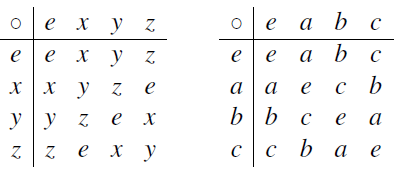Groups: In the remainder of the notes we will be talking about groups. A group is a structure with just one binary operation, satisfying four axioms. So groups are only half as complicated as rings! As well as being new material, this part will help you revise the first part of the course, since a lot of things work in almost exactly the same way as for rings.

Definition of a group: A group is a set G with one binary operation (which we write for now as  in infix notation1) satisfying the following four axioms (G0)–(G3):
(G0) (Closure law) For any g,h ∈ G, we have g . h ∈ G.
(G1) (Associative law) For any g,h,k ∈ G, we have (g . h) . k = g . (h . k).
(G2) (Identity law) There is an element e ∈ G with the property that ge=eg= g for all g ∈ G. (The element e is called the identity element of G.)
(G3) (Inverse law) For any element g ∈ G, there is an element h ∈ G satisfying g . h = h . g = e. (We denote this element h by g−1, and call it the inverse of g.) If a group G also satisfies the condition

(G4) (Commutative law) For any g,h ∈ G, we have g . h = h . g, then G is called a commutative group or (more often) an Abelian group.
Examples of groups: Axioms (G0)–(G4) for a group are just axioms (A0)–(A4) for a ring but using slightly different notation (the set is G instead of R, the operation is  instead of , and so on). So we get our first class of examples:
Proposition 3.1 Let R be a ring. Then R with the operation of addition is an Abelian group: the identity element is 0, and the inverse of a is −a. This group is called the additive group or R. This is not the only way to get groups from rings.
Proposition 3.2 Let R be a ring with identity, andU(R) the set of units of R. Then U(R), with the operation of multiplication, is a group. If R is a commutative ring, then U(R) is an Abelian group. This group is called the group of units of R.
Proof Look back to Lemma 2.20. Let U(R) be the set of units of R.
(G0) if u and v are units, then so is uv. So U(R) is closed for multiplication.
(G1) The associative law for multiplication holds for all elements of R (by Axiom (M1) for rings), and so in particular for units.
(G2) 1 is a unit. It clearly plays the role of the identity element.
(G3) if u is a unit, then so is u−1.
(G4) For the last part of the Proposition, if R is a commutative ring, then (M4) holds, so that uv = vu for all u,v 2 R; in particular, this holds when u and v are units.

Example If F is a field, then every non-zero element of F is a unit. So the set of non-zero elements forms an Abelian group with the operation of multiplication. This is called the multiplicative group of the field.

Example A matrix A is a unit in Mn(F), where F is a field, if and only if det(A) ≠ 0. So the set of matrices with non-zero determinant is a group. This
group is called the general linear group, and written GL(n,F). If n > 1, this group is not Abelian.
Direct product This construction corresponds to the direct sum for rings. Let G1 and G2 be groups. The direct product G1×G2 is defined as follows:
• the set of elements is the Cartesian product (which is also denoted by G1× G2), the set of all ordered pairs (g1,g2) with g1∈G1 and g2 ∈ G2;
• the group operation is “componentwise”, that is, (g1,g2) . (h1,h2) = (g1 . h1,g2 . h2).

It is an exercise to prove that it is a group.
Cayley tables As with any binary operation, the group operation can be represented by giving an operation table. In the case of a group, the operation table is
usually called the Cayley table of the group. In principle, given the Cayley table of a finite group, we could check that all the group axioms are satisfied. Here, for example, are the Cayley tables of two groups each with four elements.Each group is Abelian, as we can see because the tables are symmetric. These two groups are obviously different: in the second group, each element is equal to its inverse, whereas this is not true in the first group. (When we come to define isomorphism, we will say that the two groups are not isomorphic.)
In fact, these groups are the additive groups of the rings Z/4Z and GF(4) respectively.